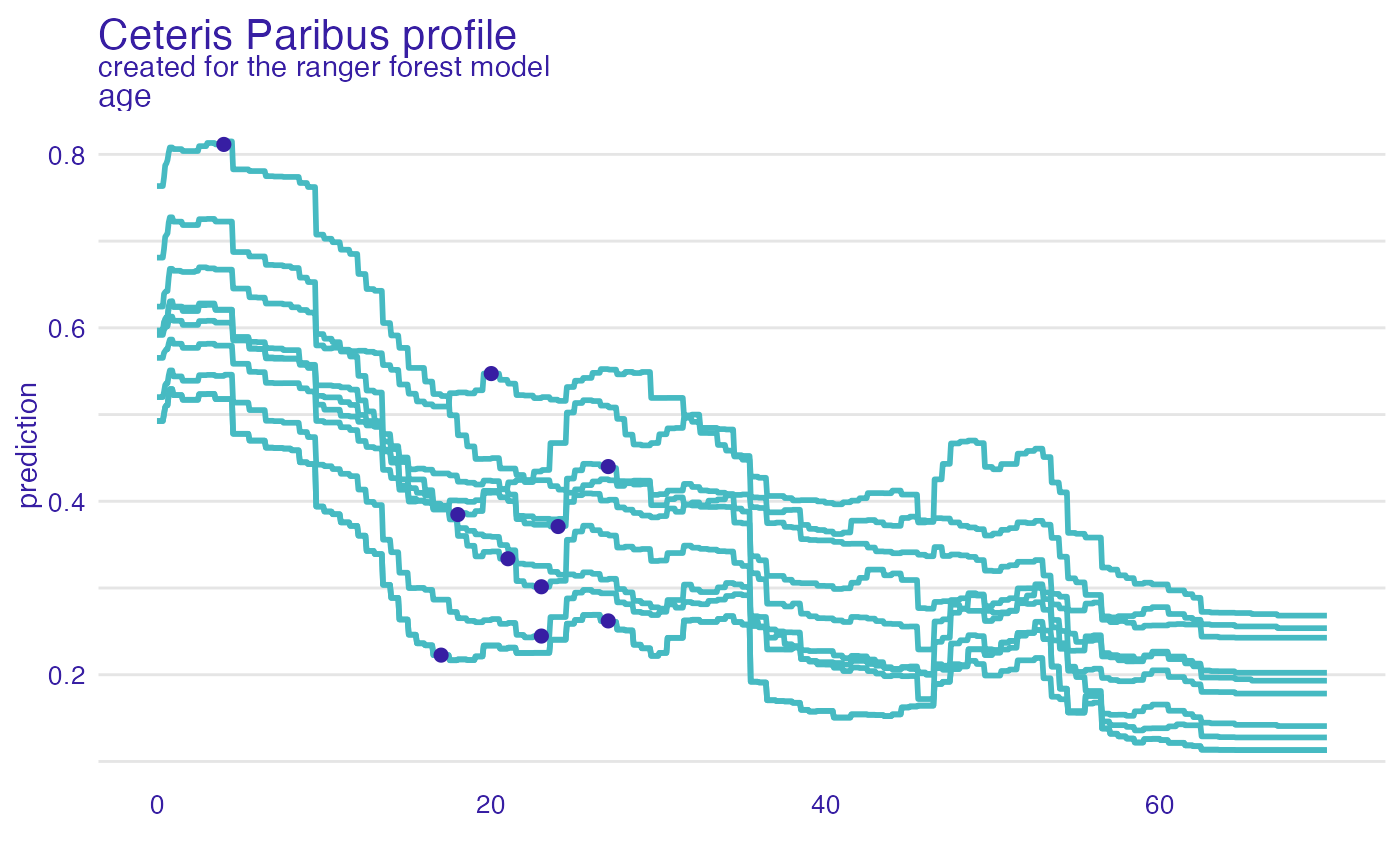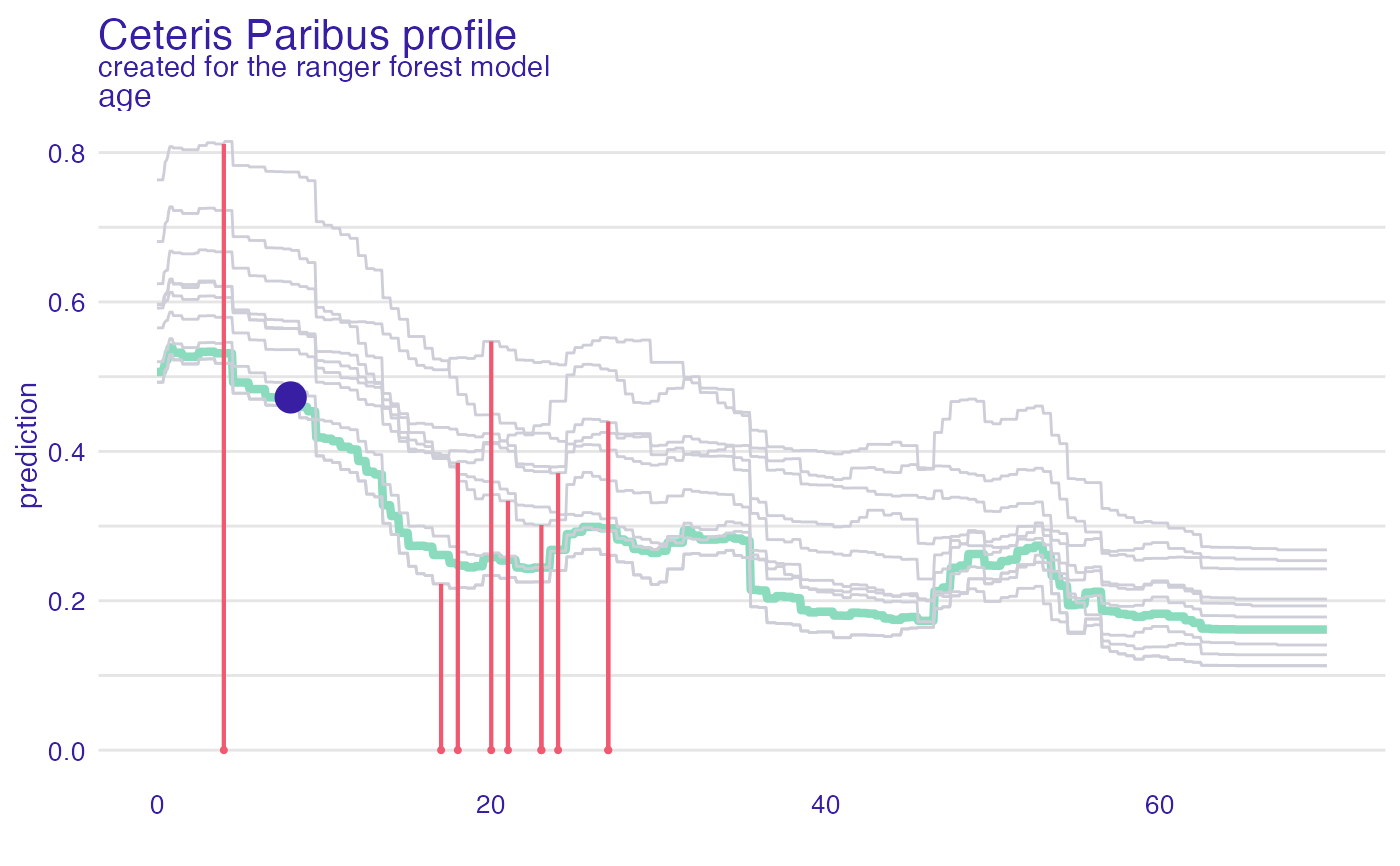Function show_residuals adds a layer to a plot created with plot.ceteris_paribus_explainer for selected observations. Note that the y argument has to be specified in the ceteris_paribus function.

show_residuals(
x,
...,
size = 0.75,
alpha = 1,
color = c(TRUE = "#8bdcbe", FALSE = "#f05a71"),
variables = NULL
)

## Arguments

x a ceteris paribus explainer produced with function ceteris_paribus(). Note that y parameter shall be supplied in this function. other explainers that shall be plotted together a numeric. Size of lines to be plotted a numeric between 0 and 1. Opacity of lines a character. Either name of a color or name of a variable that should be used for coloring if not NULL then only variables will be presented

## Value

a ggplot2 layer

Explanatory Model Analysis. Explore, Explain, and Examine Predictive Models. https://ema.drwhy.ai/

## Examples

library("DALEX")
library("ingredients")
library("ranger")

johny_d <- data.frame(
class = factor("1st", levels = c("1st", "2nd", "3rd", "deck crew", "engineering crew",
"restaurant staff", "victualling crew")),
gender = factor("male", levels = c("female", "male")),
age = 8,
sibsp = 0,
parch = 0,
fare = 72,
embarked = factor("Southampton", levels = c("Belfast", "Cherbourg", "Queenstown", "Southampton"))
)

# \donttest{
model_titanic_rf <- ranger(survived ~., data = titanic_imputed, probability = TRUE)

explain_titanic_rf <- explain(model_titanic_rf,
data = titanic_imputed[,-8],
y = titanic_imputed[,8],
label = "ranger forest",
verbose = FALSE)

johny_neighbours <- select_neighbours(data = titanic_imputed,
observation = johny_d,
variables = c("age", "gender", "class",
"fare", "sibsp", "parch"),
n = 10)

cp_neighbours <- ceteris_paribus(explain_titanic_rf,
johny_neighbours,
y = johny_neighbours\$survived == "yes",
variable_splits = list(age = seq(0,70, length.out = 1000)))

plot(cp_neighbours, variables = "age") +
show_observations(cp_neighbours, variables = "age")cp_johny <- ceteris_paribus(explain_titanic_rf, johny_d,
variable_splits = list(age = seq(0,70, length.out = 1000)))

plot(cp_johny, variables = "age", size = 1.5, color = "#8bdcbe") +
show_profiles(cp_neighbours, variables = "age", color = "#ceced9") +
show_observations(cp_johny, variables = "age", size = 5, color = "#371ea3") +
show_residuals(cp_neighbours, variables = "age")# }